Select Page

# CBSE 12 Science Answers for MCQ Maths Three Dimensional Geometry in English

CBSE 12 Science Answers for MCQ Maths Three Dimensional Geometry in English to enable students to get Answers in a narrative video format for the specific question.

Expert Teacher provides CBSE 12 Science Answers for MCQ Maths Three Dimensional Geometry through Video Answers in English language. This video solution will be useful for students to understand how to write an answer in exam in order to score more marks. This teacher uses a narrative style for a question from Three Dimensional Geometry not only to explain the proper method of answering question, but deriving right answer too.

Please find the question below and view the Answer in a narrative video format.

Question:

## Similar Questions from CBSE, 12th Science, Maths, Three Dimensional Geometry

Question 1 : Find the distance between the point (-1, -5, -10) and the point of intersection of lineand plane

Question 2 : If the cartesian equations of a line arewrite the vector equation for the line. (View Answer Video)

Question 3 : Find the angle between lineand the plane 2x - y + 2z -13 = 0. (View Answer Video)

Question 4 : Find the co-ordinates of the point, where the lineintersects the plane x-y+z-5=0. Also find the angle between the line and the plane. (View Answer Video)

Question 5 : Find the distance of the pointfrom the plane 3x + y - z + 2+0 measured parallel to the line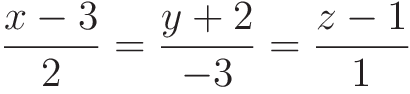Also, find the foot of the perpendicular from the given point upon the given plane. (View Answer Video)

### Inverse Trigonometric Functions

Question 1 :is equal to : (View Answer Video)

Question 2 : IfFind the values of x. (View Answer Video)

Question 3 : Write the principal value of(View Answer Video)

Question 4 : Evaluate :(View Answer Video)

Question 5 :What value do you observe in real life scenario ? (View Answer Video)

### Differential Equations

Question 1 : Determine degree of(View Answer Video)

Question 2 : If m and n are the order and degree, respectively of the differential equationthen write value of m+n. (View Answer Video)

Question 3 : Find the particular solution of the differential equationgiven that y = 1 when x = 0. (View Answer Video)

Question 4 : Find the general solution of differential equation(View Answer Video), Find P(E or F).         (View Answer Video)
Question 3 : If P(A)=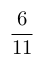, P(B)=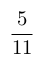and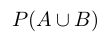=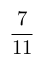, find. (View Answer Video)